# WaveNet: Increasing reception field using dilated convolution

WaveNet is an generative model for audio waveform, which was proposed by Google DeepMind through [WaveNet: A Generative Model for Raw Audio] about a year(Late 2016) ago.

Essentially waveform data is of high frequency and the sample size amounts to at least 16,000 samples per second for a reasonable quality. To deal with this issue the authors of WaveNet introduced a couple of tricks but still needs huge network to model complex pattern of wave forms. After years of engineering to make the network compact, it bases one of the Google’s AI service, Cloud-text-to-speech.

## Implementation is not easy always

Before we dive into implementation, I would like note that implementation is quite different from just reading paper to grab main idea. WaveNet is also quite hard to implement only with information presented in the paper itself. This is partly because the lack of space allowed for an article in journals and partly because the authors decided not to disclose every detail intentionally or mistakingly. Or it could be just errors in figures, which confuses researchers making them spend tons of time in wrong place. Personally, reading and understanding the main idea of the paper is not a big deal to me, but I feel frustrated almost every time when I need to implement the algorithm. As far as I know, I am not the only one who suffers like this. Trust me. Because of that, recently, many AI research groups have both research and technical units to speed up their research. In this post, I will go over some detail that is necessary for the implementation, which may not presented clear enough in the paper.

# WaveNet can be huge, we need tricks for it

The size of waveform data depends on both of sampling rate and bit depth. The sound sampled with 44.1 kHz has 44,100 slices every second, which means we have 44,100 data points per second.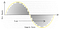http://wp.tweakheadz.com/wp-content/uploads/2013/02/bit-depth-vs-sample-rate-tweakheadz-dot-com.png

In the case of stereo sound, it doubles the size of course. 16 bit sound has bit depth of 65,536, that means we need to predict one of 65,536 possible outputs at a given time points, which is much more difficult than usual classification problem. WaveNet introduced two key tricks to address those issues.

## Dilated Convolution

Since we make and hear sound in time order, it can be considered as an extremely correlated time series data. Due to its high frequency, it also can be thought of as long dependency data since many samples are involved even for a very short sound. To address this, waveforms needs to consider many prior samples to predict the next value. To increase reception field exponentially with linearly increasing number of parameters, the authors applied dilation convolutions as is depicted in the diagram below.

Assuming kernel of size 2 (which only considers the relation between two data points) with 16 channels (which capture 16 different properties of the waveform) , 4 Layers of dilated convolutions contains (16 * 2 * 4 = 128) parameters. It compares to (16 * 2 * 15 = 480) of parameters needed to cover 16 receptive field with the regular 1D convolution kernel of size 2, since we need 15 layers to model relationship between 16 values. The gap between dilated convolution and regular convolution grows quadratically to the number of values to cover. This idea is even more developed in [fast WaveNet] for better speed.

## Companding transformation

As is mentioned above, 16-bit waveform can have one of 65,536 distinct values for a given time point. In data generation process, we need to predict one of 65,536 possibilities and it is too difficult to do so. The authors pay attention to the fact that people are more sensitive to changes in small sound but not that much in large sound. So they consider the values around zero more carefully than those around 1 (after standardization) by introducing a non-linear transformation, called companding transforms and is given as follows:

The transformation stretches the gap between numbers around zero and squeezes the numbers around 1 or -1. By doing this, we shrank the original problem into that with 256 categories without losing too much information. The following plot shows the transformation after rescaling the output to fit in between 0 and 255.

Of course, we need to transform back to the original scale after the prediction to generate a relevant waveform using the reverse transformation.

# Architecture

After pretty much time spending on the material for ‘WaveNet’, I found that there are not many intuitive diagram that captures the entire structure of WaveNet. The animation given in official webpage posted by Google DeepMind, has a nice illustration on the mechanism behind dilated convolution but not enough to understand the entire structure.

I tried to put everything in a single diagram at the beginning, but I realized that it was almost impossible at the end of the day. So I broke the structure down into a couple of parts. Let us start with input data structure.

## How to process input data?

Assuming that we have a waveform of length, n = 82,081 (The size of data from the example code), data are given as a list of integers. Those integers range from 0 to 255 after the transformation. (Actually the maximum is 129)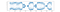Sample data of length 20,000 after transformation

We perform a mini-batch update for the parameters of network. To construct mini-batch, we first select a random location (blue dot) and take 20,000 values from it. The input should be one-hot encoded which is shown at the bottom of the diagram below. In this case, our mini-batch size is 17,953 in this case and will explain why we have this number in the later of this article.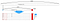Data Preprocess

We have to understand residual block first to see the architecture.

## Residual block?

Residual block can be thought of as a building block for WaveNet . There are as many blocks as the number of dilation layers. For each residual block, the output of the previous block will be fed into the next one. By doing this, local features in low dilation layers are accumulated sequentially to capture dependency between the data points at distance. Let take a look at the inside block.

A residual block produces two outputs:

1 . Feature map from the previous residual block, which will be used as an input for the next one.

2. Skip connection, which will be used to calculate loss function for the batch after the aggregation.

Inside residual block, inputs will be running through two separate 2x1 dilated convolution layers. One is for gate and the other is for filter. They borrowed (not actually borrowed since it’s their idea as well) gated activation unit idea from PixelCNN(van den Oord et al., 2016b). Filter and gates will be multiplied in element-wise¹ ((1) in the diagram). Now, we can easily understand this operation written in the following equation more easily from the diagram.Equation (2) from the paper

Gated activation output, z, is summed with the original input to make z a residual² . For your information, it is well-known that modeling residual allows for the network going deeper and stable. This idea first introduced in [Identity Mappings in Deep Residual Networks] as far as I know. Correct me if I am wrong. For more information, you can read this.

Part (3)’s in the diagram refer to 1x1 convolution layers, which are used to change number of layers without affecting the size of feature map usually to save the number of parameters. It is first used by Google Inception network proposed in [Going Deeper with Convolutions] and is now widely used in the recent developments in image/vision area.

## How to weave residual blocks to get losses

There is a korean anecdote, “Only when glass beads are strung together then it becomes a treasure”, meaning that Nothing is complete unless you put it into the final shape. It’s time to link residual blocks to build a graph leading input information toward loss function, which measures discrepancy between prediction and ground truth. Of course, there are many illustrations available on the web, I did draw a diagram myself to enhance understanding. I made the following assumptions for the diagram.

• We take 20,000 samples for training.
• There are 10 residual blocks, with [1, 2, 2¹, 2², …, 2⁹] (We do this twice in example code)
• 1x1 convolutions for residual block is 24, that for skip connection is 128.
• There are 256 distinct values for the response (after companding transformation)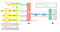Connections between residual blocks

Please take a look at (1) part in the above diagram. As input data goes through residual blocks, the outputs get shorter and shorter since dilated convolution skips 2⁰, 2², …, 2⁹ samples when it convolves with filter of size 2. Finally, we get 18,976 elements for the top residual block in this example.

Since we will use the outputs from the 10th residual block to predict the next value, we only have 18,976 complete predictions. That means the first 1,023 values (out of 19,999) don’t have enough data to reach to the final residual block. Therefore, as is depicted in (2) part from the above figure, we take the sum over skip connections from 10 residual blocks only for the last 18,976 values. After that, we will have the output of size 18,976 x 128. Another two 1x1 convolution layers shape the output finally into 18,976 x 256, where 256 represents the number of categories to predict. Part (3) denotes the way we get the loss function based on 18,976 last observed values that lies in the position from 1,025 to 20,000.

In the example code, we have 17, 953 last values (still out of 20,000) as ground truth for loss calculation.

# Implementation details

Finally, we are ready to write down the code for network. The entire implementation can be found at the [github] maintained by one of my enthusiastic colleagues, Seung Hwan Jung.

## Defining network

The entire network is defined with WaveNet class. In constructor, we define elements such as filters, gates, 1x1 convolutions for skip connection and outputs.

We have those elements as many as dilation layers, which is defined in `self.dilations`. The results from residual blocks are fed into `self.conv_post_1` and `self.conv_post_2,` in respect, after the aggregation.

How we weave residual blocks are written in `self.forward` method as follows:

`self.preprocess` method apply a convolution layer to prepare one-hot encoded input data suited for the following residual blocks.

Prior to this, we apply one-hot encoding to input data as well. He defined `One_Hot` class for it.

It iterates through to the number of dilation layer to define residual blocks and sum skip connections up to update parameters from layers. Here int `self.postprocess`, we apply the following convolution layers and softmax layer as well.

Residual block is defined in `self.residue_forward` method given below.

Gated filters and skip scales are calculated before being applied to 1x1 convolutions. The input itself is added to the output of residual scale at the very last step. As is said, it produces two outputs, one for the next residual block and the other for the graph that leads to loss function.

## Generating waveform

Generation seems to be relatively easier. Just apply the following code. It passes the result from one time point to next sequentially.

# Results

Since it involves to much operations happening sequentially, it takes very long time to generate just about 1 second of waveform with this example code. It is the point that needs to be addressed somehow in [Fast Wavenet Generation Algorithm] and [Parallel WaveNet: Fast High-Fidelity Speech Synthesis]. From loss trajectory, we can see that losses decreases rapidly within the first 50 iterations and then fluctuate in between 0 and 20,000.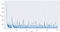Loss trajectory

We can transform back to the original sound and it seems to be reasonable when I listen to.

Written by

## KION KIM

#### Applied Scientist, @AI Center, SK Telecom

Welcome to a place where words matter. On Medium, smart voices and original ideas take center stage - with no ads in sight. Watch
Follow all the topics you care about, and we’ll deliver the best stories for you to your homepage and inbox. Explore
Get unlimited access to the best stories on Medium — and support writers while you’re at it. Just \$5/month. Upgrade